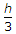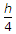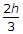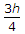# Civil Engineering - Applied Mechanics - Discussion

Discussion Forum : Applied Mechanics - Section 1 (Q.No. 46)
46.
The c.g. of a thin hollow cone of height h, above its base lies on the axis, at a height ofExplanation:
No answer description is available. Let's discuss.
Discussion:
10 comments Page 1 of 1.

Mali alpesh k said:   9 months ago
Centre of gravity of a thin hollow cone = 1/3
Centre of gravity of a solid cone = 1/4.

Onkar k said:   4 years ago
Hollow cone = h/3.
Solid cone = h/4.

Tirth shete said:   4 years ago
Cone:h/4.
Hollow cone : 2h/3.

Shashu said:   5 years ago
The correct Answer must be option A.

Ashwini said:   5 years ago
It Should be h/3.

Venkat said:   6 years ago
Hollow: h/3.
Solid: h/4.
From base.

Samprit said:   6 years ago
Why not h/4 is there any differencee in C.G. of hollow and solid circular cone?

Krp said:   8 years ago
When the cone is positioned upright, standing on its tip we can find the center of mass by the formulas,
x' = ∫(x*da)/∫(da).
y' = ∫(y*da)/∫(da).
---with the limits from,
x = 0 to x = b(base).
y = 0 to y = h(height).

By intuition center of mass along x = 0.

We can represent the equation of the cone as y = mx, where m is the slope.

So finding the center of mass along y we find,
∫yda = ∫y*2πxdy, since da=2πxdy from infinitesimal area A = 2πrh.
= 2π∫(y^2)dy/m.
= 2π(y^3)/3m evaluated from 0 to h.
= 2πh^3/3m, we can find m from y = mx ---> h = mb so m=h/b.
= 2πb(h^2)/3.

Then we have to find ∫da,
∫da = ∫2πxdy.
= ∫2πydy/m.
= π(h^2)/m.
= πbh.

so y' = ∫(y*da)/∫(da) = 2πb(h^2)/3πbh = 2h/3.
This means the center of mass along the vertical axis of the cone is 2h/3 when the cone is STANDING ON ITS TIP.
y = 2h/3 and x = 0.

Danesh said:   9 years ago
From top its 2h/3 and from base it is h/3.

Neetha said:   10 years ago
How come 2h/3, It should be h/3?